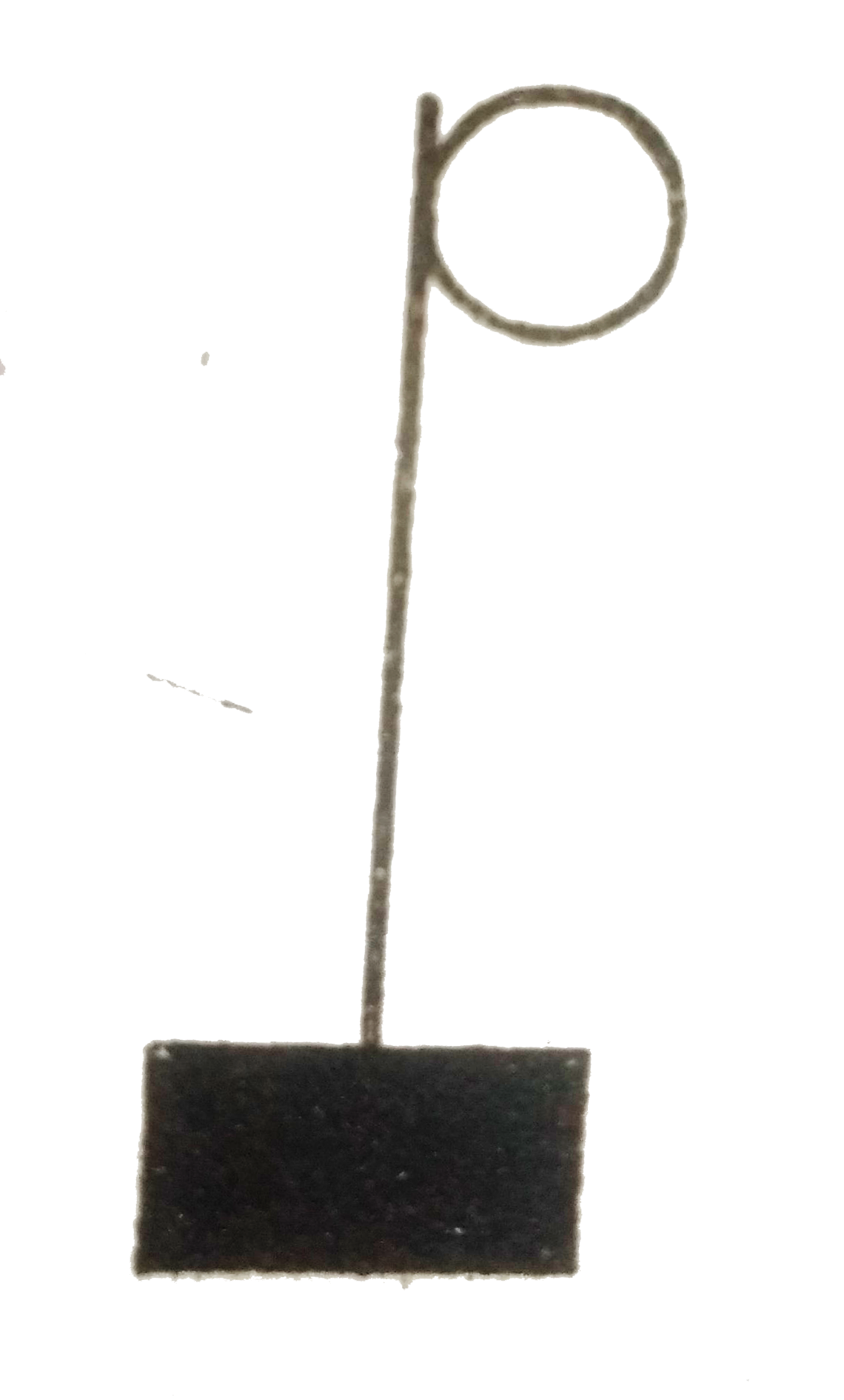# A block hangs from a string wrapped on a disc of radius 20 cm free to rotate about its axis which is fixed in a horizontal position. If the angular sp

32 views
in Physics
closed
A block hangs from a string wrapped on a disc of radius 20 cm free to rotate about its axis which is fixed in a horizontal position. If the angular speed of the disc is 10 rad/s at some instant, with whtat speed is the block going down at that instant?

by (75.0k points)
selected

The block is moving on the rim of the pulley. The pulley is moving at omega=10rad/s
Therefore the radius of the pulley
=20 cmTherefore linear velocity on the rim
=tangential velocity =romega
=10xx20=200cm/s
=2m/s
Therefore velocity of the block
=2m/s